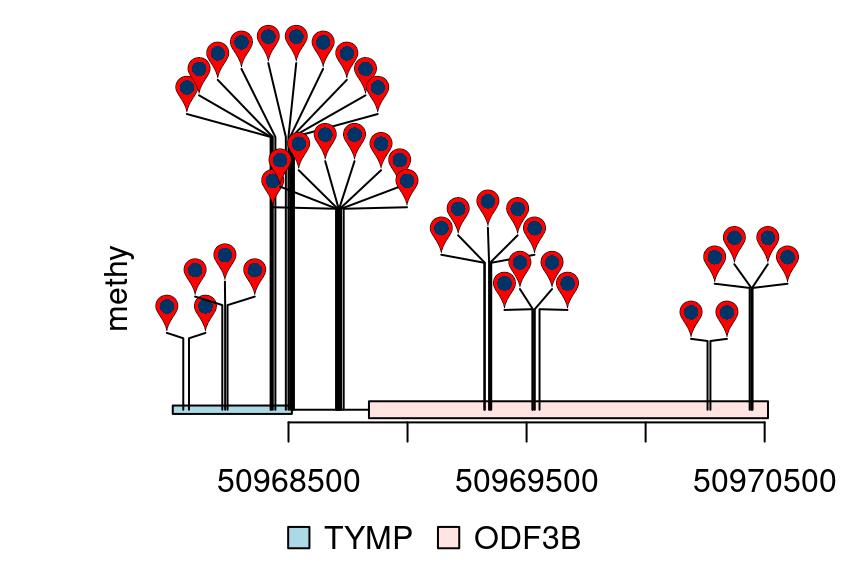# Dandelion Plot

Sometimes, there are as many as hundreds of SNPs invoved in one gene. Dandelion plot can be used to depict such dense SNPs. Please note that the height of the dandelion indicates the desity of the SNPs.

``````library(trackViewer)
library(TxDb.Hsapiens.UCSC.hg19.knownGene)
library(org.Hs.eg.db)
library(rtracklayer)
methy <- import(system.file("extdata", "methy.bed", package="trackViewer"), "BED")
gr <- GRanges("chr22", IRanges(50968014, 50970514, names="TYMP"))
trs <- geneModelFromTxdb(TxDb.Hsapiens.UCSC.hg19.knownGene,
org.Hs.eg.db,
gr=gr)
features <- c(range(trs[]\$dat), range(trs[]\$dat))
names(features) <- c(trs[]\$name, trs[]\$name)
features\$fill <- c("lightblue", "mistyrose")
features\$height <- c(.02, .04)
dandelion.plot(methy, features, ranges=gr, type="pin")``````## Change the type of Dandelion plot

There are one more type for dandelion plot, i.e., type “fan”. The area of the fan indicates the percentage of methylation or rate of mutation.

``````methy\$color <- 3
methy\$border <- "gray"
## Score info is required and the score must be a number in [0, 1]
m <- max(methy\$score)
methy\$score <- methy\$score/m
dandelion.plot(methy, features, ranges=gr, type="fan")``````